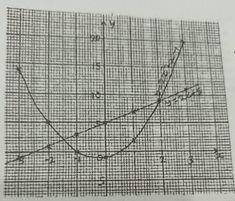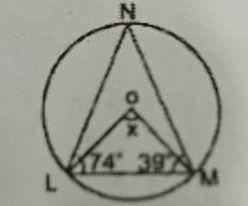### Mathematics Past Questions

4276The graph of the equations y = 2x + 5 and y = 2x$$^2$$ + x - 1 are shown. Use the information above to answer this question.

Find the point of intersection of the two graphs.

• A. (2.0, 9.0) and (-1.5, 2.0)
• B. (2.0, 8.5) and (-1.5, 2.0)
• C. (2.0, 8.0) and (-1.5, 2.5)
• D. (2.0, 7.5) and (-1.5, 2.5)
WAEC 2020
4277The graph of the equations y = 2x + 5 and y = 2x$$^2$$ + x - 1 are shown. Use the information above to answer this question.

If x = -2.5, what is the value of u on the curve?

• A. y = 8.0
• B. y = 8.5
• C. y = 9.0
• D. y = 9.5
WAEC 2020
4278

If (x + 2) is a factor of x$$^2$$ +px - 10, find the value of P.

• A. 3
• B. -3
• C. 7
• D. -7
WAEC 2020
4279In the diagram, O is the centre of the circle. If < NLM = 74$$^o$$, < LMN = 39$$^o$$ and < LOM = x, find the value of x.

• A. 134$$^o$$
• B. 126$$^o$$
• C. 113$$^o$$
• D. 106$$^o$$
WAEC 2020
4280

Find the least value of x which satisfies the equation 4x = 7(mod 9)

• A. 7
• B. 6
• C. 5
• D. 4
WAEC 2020